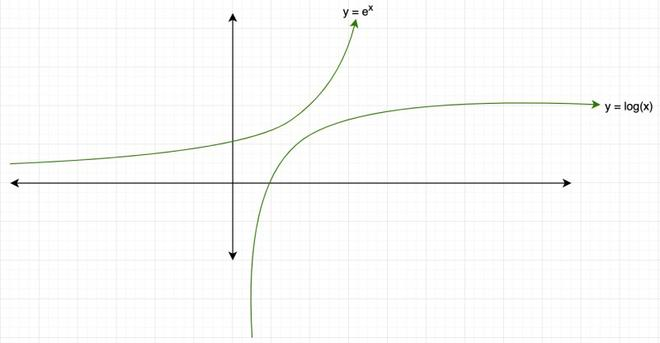GeeksforGeeks App
Open AppBrowser
Continue

# Derivative of Exponential and Logarithmic Functions

Exponential and Logarithmic functions are a class of functions that are used a lot in different areas of sciences. Exponential functions increase very rapidly and logarithmic functions tend to saturate themselves as the input values increase. In the figure below, it can be noticed that as the power of x increases, functions start to grow faster and the graph becomes steeper. Exponential functions come in a similar class of functions.

The figure below represents the graph of a logarithmic and exponential function.### Derivative of Exponential Function

Let’s start off by looking at the exponential function,

y = e

For its differentiation, normal power use that is used usually won’t work. So, let’s derive the derivative of this using limits. The derivative of a function using limits is given by,Now this last limitis exactly the definition of above derivative f'(x) at x = 0, i.e f'(0). Therefore, the derivative becomes,

f'(x) = bxf'(0) = bx

So, in case of natural exponential functions, f(x) = ex

Note: In general exponential cases, for example, y = bx, where b is a real number. The derivative for this kind of function isQuestion 1: Differentiate f(x) = 4ex – 5x

The derivation of ex will remain ex, the derivative of 5x will become 5xln(5) as explained above.

Therefore, f'(x) = 4ex – 5xln(x)

Question 2: DifferentiateHere, following Quotient Rule, differentiate the function g(x):Question 3: Find the value of F'(x) at x=0 when f(x) = 7x + 2ex

Differentiating: f'(x) = 7xln(7) + 2ex

at x=0, f'(0) = 70ln(7) + 2e0

= ln(7) + 2

= 3.945

### Derivative of Logarithmic Function

Now, let’s look at how the derivatives for the logarithmic function are calculated. Notice the fact that these functions are actually inverses of each other.

Note: If two functions are inverses of each other then,It is a known fact that natural exponential and natural log are the inverses of each other. We can use the above property to find the derivative for the logarithmic function.

Let’s say f(x) = ex and g(x) = logex.Let’s look at some examples on derivatives of both of these functions,

Question 1: Differentiate: y(x) = x5 – exln(x)

Differentiating using chain rule:Question 2: Calculate the derivatives for

(i) e-x              (ii) sin(logx)              (iii) ecos(x)

(i) Let y = e-x, to find the differentiation of this function we need to use chain rule.(ii) y = sin(logx). It also requires chain rule for differentiation.(iii) y = ecos(x)Question 3: Calculate the derivative f(x) = 3ex + 10x3log(x).